# Grade - math word problems

#### Number of examples found: 5346

• Passenger car and truckFrom Kutna Hora left at 11:00 clock a truck at 60 km/h. At 12:30 behind him started passenger car at average speed 80 km/h. At what time and how far from Kutna Hora overtake a passenger car truck?
• Shoes and slippers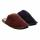Shoes were three times as many as slippers. If shoes were cheaper by 120, it would be twice as expensive as slippers. How much cost shoes and how much slippers?
• Medicine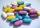We test medicine on 6 patients. For all drug doesn't work. If the drug success rate of 20%, what is the probability that medicine does not work?
• Cars motion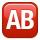From point A car start at the speed of 90 km/h. Against it departs from the point B at the same time another car at 60 km/h. For how long and where they met if the distance between A and B is 180 km?
• Rectangular trapezoidCalculate the content of a rectangular trapezoid with a right angle at the point A and if |AC| = 4 cm, |BC| = 3 cm and the diagonal AC is perpendicular to the side BC.
• KLMNIn the trapezoid KLMN is given this informations: 1. segments KL and MN are parallel 2. segments KL and KM has same length 3. segments KN, NM and ML has same length. Determine the size of the angle KMN.
• Circular motionMass point moves moves uniformly in a circle with radius r = 3.4 m angular velocity ω = 3.6 rad/s. Calculate the period, frequency, and the centripetal acceleration of this movement.
• HexagonThere is regular hexagon ABCDEF. If area of the triangle ABC is 22, what is area of the hexagon ABCDEF? I do not know how to solve it simply....
• TetrahedronCalculate height and volume of a regular tetrahedron whose edge has a length 4 cm.
• VAT lottery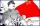Father earns 993 euro a month, mother 918 euro per month. Calculate what amount they paid as value added tax (VAT) to the government if the VAT rate is 20%. Assume that the family will spend their entire monthly income.
• ObserverThe observer sees straight fence 100 m long in 30° view angle. From one end of the fence is 102 m. How far is it from the another end of the fence?
• LogicA man can drink a barrel of water for 26 days, woman for 48 days. How many days will a barrel last between them?
• Right ΔA right triangle has the length of one leg 11 cm and length of the hypotenuse 61 cm. Calculate the height of the triangle.
• Energy consumption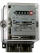The device is connected to 230V and draws 3.5A current. Power consumption is 1932kJ. How many minutes has this device been in operation?
• An equilateralAn equilateral triangle is inscribed in a square of side 1 unit long so that it has one common vertex with the square. What is the area of the inscribed triangle?
• Self-oscillation period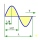The water in the vessel carried by the boy has a self-oscillation period of 0.8 s. What is the size of the boy's movement speed when the length of the boy's step is 60 cm? Give the result in m/s.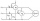Two wattmeters are connected to measuring power in a 3 phase balanced load. Determine the total power and power factor if the two wattmeters read 1000 watts each (1) both positive and (2) second reading is negative
• Two cubesThe surfaces of two cubes, one of which has an edge of 22 cm longer than the second are differ by 19272 cm2. Calculate the edge length of both cubes.The regular quadrangular pyramid has a base length of 6 cm and a side edge length of 9 centimeters. Calculate its volume and surface area.A probability of disease A on the island of Utopia is 40%. A probability of occurrence among the men of this island, which make up 60% of all the population (the rest are women), is 50%. What is the probability of occurrence of A disease among women on Ut